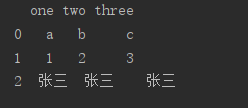# How to Solve attributeerror: ‘list’ object has no attribute ‘shape‘

Explanation:

AttributeError: ‘list’ object has no attribute ‘shape’

Property error: the ‘list’ object does not have the property ‘shape’

resolvent:

Use numpy or panda np.array Or dataframe has shape, which can be multi-dimensional, while list is one-dimensional and cannot be converted

If conversion is needed, list is converted to dataframe. Example:

``````a = [['a', 'b', 'c'], ['1', '2', '3'], ['张三', '张三', '张三']]
df = pd.DataFrame(a, columns=['one', 'two', 'three'])
print(df)``````List to numpy example:

``````a = [['a', 'b', 'c'], ['1', '2', '3'], ['张三', '张三', '张三']]
data = np.array(a)
print(data)``````Note: shape is a dimension. Only data frame and matrix have dimensions, while list is a slice single dimension